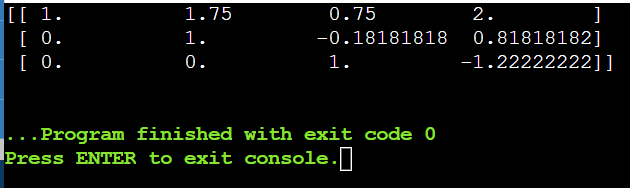# row_echelon_form method using elementary operator

## Question

import numpy as np
class Matrix:

def __init__(self matrix):

self._matrix = matrix

def type_I_elementary_row_operator (self, i, j):

temp = self._matrix[i].copy()
self._matrix[i] = self._matrix[j]
self._matrix[j] = temp

def type_II_elementary_row_operator (self, number, row):

self._matrix[row] = self._matrix [row] * number

def type III elementary_row_operator (self, number, row1, row2):

temp = self._matrix [row1]. copy() * number
self._matrix [row2] -= temp

Write row_echelon_form method using the elementary operator.

## Summary

In this question, we have to write a row_echleon_form method using the elementary operator. There is a direct function with the name rref() of Matrix class in python in sympy package.
We have given the code under explanation.
In this question, majorly we convert a matrix to row-echelon form using elementary transformations.
There are certain functions for row transformations already defined in python in the question given.
For the matrix given:
A = 4 7 3 8
8 3 8 7
2 9 5 3
For the above matrix the row echelon form is:
1   1.75   0.75                2
0   1       -0.18181818    0.81818182
0   0        1                     -1.22222222

## Explanation

There is a direct function with the name rref() of Matrix class in python in sympy package. We can directly use it to find the row-echelon form of a matrix as shown below.

The class named Matrix is implemented with a constructor and a private matrix attribute. To perform basic elementary operations, we have defined three functions namely, type_I_elementary_row_operator, type_II_elementary_row_operator and type_III_elementary_row_operator.
We have defined the function row_echelon to convert a matrix to its row-echelon form.

In this function, if the matrix is empty, it is already in echelon form, so we return the same matrix. We check for a non-zero element in the first column, if all elements of 1st column are zeroes, then we perform ref on 2nd column. Eventually, add the first column. if there is no non-zero element in the first row, we swap the row containing non-zero element with 1st-row#, we divide the first row entirely with the first element in that row. All the subsequent rows will be subtracted from the first row. Also, we will multiply with the first column corresponding element. Then, we do the ref on the matrix from the 2nd row and 2nd column. Finally, add the first row and first column.

## Code

Below is the code for row_echelon_form method.

import numpy as np

class Matrix:
def __init__(self, matrix):
self._matrix=matrix

def type_I_elementary_row_operator(self, i, j):
temp=self._matrix[i].copy()
self._matrix[i]=self._matrix[j]
self._matrix[j]=temp

def type_II_elementary_row_operator(self, number, row):
self._matrix[row]=self._matrix[row]*number

def type_III_elementary_row_operator(self, number, row1, row2):
temp=self._matrix[row1].copy()*number
self._matrix[rows2]-=temp

def row_echelon(self, matrix):
""" Return Row Echelon Form of matrix A """

# if the matrix is empty, it is already in echelon form
r, c = matrix.shape
if r == 0 or c == 0:
return matrix

# to check for a non-zero element in first column
for i in range(len(matrix)):
if matrix[i,0] != 0:
break
else:
# if all elements of 1st column are zeroes
# ref is performed on 2nd column
X=matrix
T = self.row_echelon(X[:,1:])
return np.hstack([matrix[:,:1], T])

# if there is no non-zero element in the first row
# we swap the row containing non-zero element with 1st row
if i > 0:
type_I_elementary_row_operator(i,0)

# we divide the first row entirely with first element in that row
matrix = matrix / matrix[0,0]

# all subsequent rows will be subtracted with first row
# also, will be multiplied with the first column corresponding element
matrix[1:] -= matrix * matrix[1:,0:1]

# we do the ref on the matrix from the 2nd row and 2nd column
B = self.row_echelon(matrix[1:,1:])

#add first row and first column
return np.vstack([matrix[:1], np.hstack([matrix[1:,:1], B]) ])

A = np.array([[4, 7, 3, 8],
[8, 3, 8, 7],
[2, 9, 5, 3]], dtype='float')

obj=Matrix(A)
print(obj.row_echelon(A))


## OutputAlso read, What is __init__.py for?